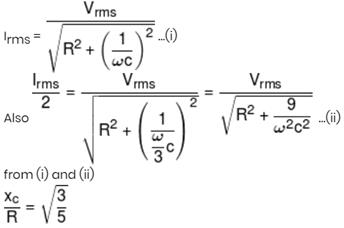Courses

# Physics Test 5 - Magnetic Effect, Magnetism, Electromagnetic Induction And Alternating Current

## 25 Questions MCQ Test Mock Test Series for JEE Main & Advanced 2022 | Physics Test 5 - Magnetic Effect, Magnetism, Electromagnetic Induction And Alternating Current

Description
This mock test of Physics Test 5 - Magnetic Effect, Magnetism, Electromagnetic Induction And Alternating Current for JEE helps you for every JEE entrance exam. This contains 25 Multiple Choice Questions for JEE Physics Test 5 - Magnetic Effect, Magnetism, Electromagnetic Induction And Alternating Current (mcq) to study with solutions a complete question bank. The solved questions answers in this Physics Test 5 - Magnetic Effect, Magnetism, Electromagnetic Induction And Alternating Current quiz give you a good mix of easy questions and tough questions. JEE students definitely take this Physics Test 5 - Magnetic Effect, Magnetism, Electromagnetic Induction And Alternating Current exercise for a better result in the exam. You can find other Physics Test 5 - Magnetic Effect, Magnetism, Electromagnetic Induction And Alternating Current extra questions, long questions & short questions for JEE on EduRev as well by searching above.
QUESTION: 1

Solution:
QUESTION: 2

### A closely wound flat circular coil of 25 turns of wire has diameter of 10 cm which carries current of 4 A, the flux density at the centre of a coil will be

Solution:

Flux density = 2 * π * N * i/10r
or,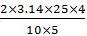= 12.56G or 1.25 * 10-3T

QUESTION: 3

### An electron is travelling along the x-direction. It encounters a magnetic field in the y-direction. Its subsequent motion will be

Solution: Because a force of q(v×B) is acted on e⁻ toward +z axis that will change the direction of e⁻ and then it will move in a circle in x-z plane
QUESTION: 4
The magnetic field due to a straight conductor of uniform cross-section of radius a and carrying a steady current is represented by
Solution:
QUESTION: 5

A cyclotron is operating at a frequency of  12 * 106 Hz. Mass of deuteron is 3.3 * 10-27 kg  and charge on deuteron is 1.6 * 10-19  induction of the neccessary magnetic field is

Solution:
QUESTION: 6

The strength of the transverse magnetic field required to bend photoelectrons of maximum kinetic energy with in a circle of radius 50 cm when light of wavelength 4000Å is incident on barium emitter is (work function of barium is 2.5 eV)

Solution:

key idea: Body performing circular motion is always acted upon by a centripetal force provided by magnetic field. If the kinetic energy of photoelectrons emitted from the metal surface is E1 and W the work function them from Einstein's photoelectric equation, we have Eh = hv -W where v is frequency and h the Planck's constant
∴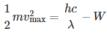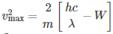Putting the numercial values from the question, we have
∴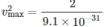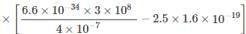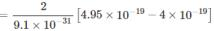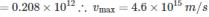A body performing circular motion is acted upon by a force directed towards its centre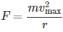Also the magnetic force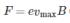On equating.
We have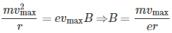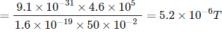QUESTION: 7
The semicircular portion (radius R) of a wire carrying current i is placed in a uniform magnetic field B. The magnetic field is perpendicular to the plane of the circle. The magnetic of the force on the wire is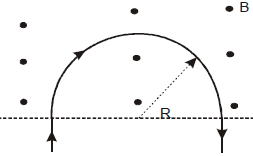Solution:
QUESTION: 8
A & B are two concentric circular conductors of centre O and carrying currents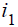and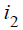as shown in the diagram. If the ratio of their radii is 1:2 and ratio of the flux densities at O due to A and B is 1:3, then the value of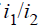will be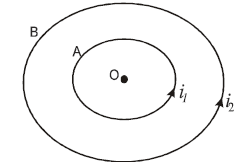Solution:
QUESTION: 9
Domain formation is the necessary feature of
Solution:
QUESTION: 10
The direction of the null points is on the equation line of a bar magnet, when the north pole of the magnet is pointing
Solution:
QUESTION: 11
Two magnets each of magnetic moment M are placed so as to form a cross at right angles to each other. The magnetic moment of the system will be
Solution:
QUESTION: 12

At a certain place, the horizontal component of earth’s magnetic field is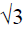times of its vertical component. the angle of dip at that place is

Solution:
QUESTION: 13

A magnet makes 30 oscillations per minute in the earth’s magnetic field. If the magnetic field is doubled. The period of oscillation of magnet is

Solution: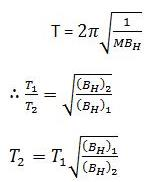n1 = 30 oscillation/min
n1 = 1/2  oscillation/s
T1 = 2s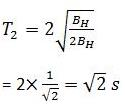QUESTION: 14
A moving conductor coil produces an induced emf. This is in accordance with
Solution:
QUESTION: 15
The varying current in a coil changes from 10 A to zero in 0.5 s. If the average emf induced in the coil is 220 V, then the self-inductance of the coil will be
Solution: Avg E= L(∆i/∆t) 220=L (10/0.5) 220=L(20) L=11H
QUESTION: 16
Two circular coils can be arranged in any of the three situations shown in the figure. Their mutual inductance will be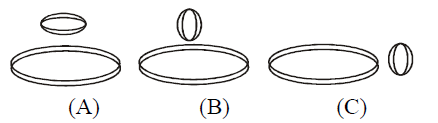Solution:
QUESTION: 17

In the circuit shown in the figure, what is the value of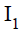just after pressing the key K?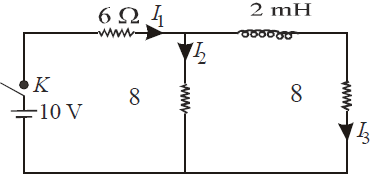Solution:
QUESTION: 18
A uniform but time-varying magnetic field B(t) exists in cylindrical region of radius a and is directed into the plane of the paper, as shown. The magnitude of the induced electric field at point P at a distance r from the centre of the circular region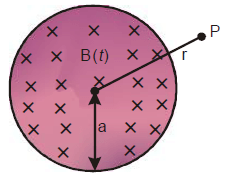Solution:
Construct a concentric circle of radius r. The induced electric field (E) at any point on the circle is equal to that at P. For this circle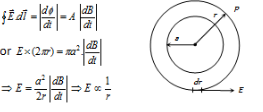QUESTION: 19
A device for generating and alternating current of a desired frequency is known as
Solution:
QUESTION: 20
In an AC circuit containing only capacitance, the current
Solution:
*Answer can only contain numeric values
QUESTION: 21

A long solenoid has 100 turns/meter and a current of 3.5 A is flowing through it. If it is filled with material of relative permeability (μr = 20) then magnetic field within the solenoid will be xπ × 10–4, find x?

Solution:

B = µ0µRni

*Answer can only contain numeric values
QUESTION: 22

A bar magnet is freely suspended in such a way that, when it oscillates in the horizontal plane. It makes 20 oscillations per minute at a place, where dip angle is 30° and 15 oscillations per minute at a place, where dip angle is 60°. Ratio of total earth's magnetic field at these two places is x : 9√3, find x :-

Solution: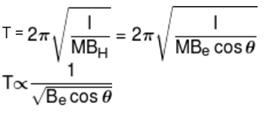*Answer can only contain numeric values
QUESTION: 23

A square loop ABCD carrying a current i, is placed near and coplanar with a long straight conductor XY carrying a current i, the net force on the loop will be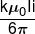Find the value of k.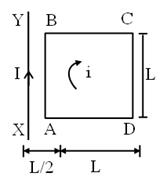Solution: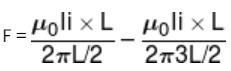*Answer can only contain numeric values
QUESTION: 24

A square metal wire loop of side 10 cm and resistance 1Ω moved with a constant velocity v in a uniform magnetic field B = 2T as shown in figure. The magnetic field is perpendicular to the plane of the loop and directed into the paper. The loop is connected to a network of resistors, each equal to 3Ω. What should be the speed of the loop in m/s so as to have a steady current of 1mA in the loop?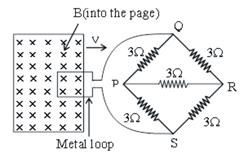Solution:

The given network PQRS is balanced Wheatstone's bridge. Hence the resistance connected in the diagonal arm PR becomes ineffective. The net effective resistance of the network is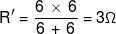The total resistance of the loop + network is
R = 1 + 3 = 4Ω
Induced current in the network,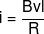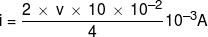v = 2 × 10–2 m/s = 2 cm/s

*Answer can only contain numeric values
QUESTION: 25

An AC source of angular frequency ω is fed across a resistor R and a capacitor C in series. The current registered is I. If now the frequency of source is changed to ω/3 (but maintaining the same voltage), the current in the circuit is found to be halved. The ratio of reactance to resistance at the original frequency ω is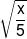then find the value of x.

Solution:

At angular frequency ω, the current in RC circuit is given by